# 从CPU cache一致性的角度看Linux spinlock的不可伸缩性(non-scalable)## 一点说明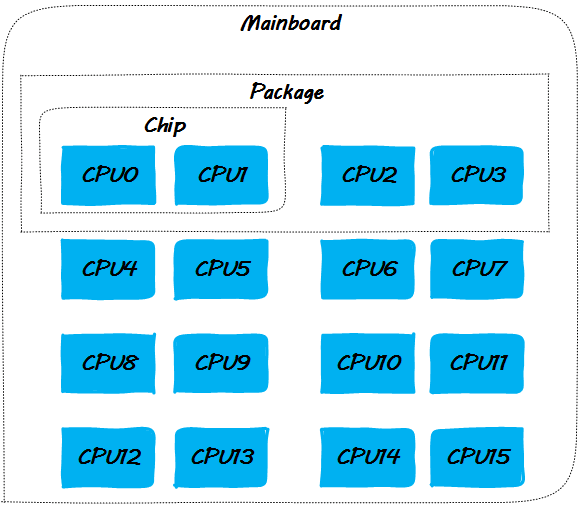• snoopy方式
这意味着cache一致性消息通过总线广播，于是就要侦听总线以在某个时机获得控制权。这种方式及其不易扩展，在CPU数目达到一定量时，总线的争抢会很严重。
参考以太网的CSMA/CD协议，会得出一样的结论，正如CSMA/CD的基于总线的以太网由于其不可扩展性进化到了交换式以太网一样，CPU间的cache一致性协议的点对点unicast实现方式最终替代了snoopy(小规模本地cache一致性依然会采用snoopy的方式)。总而言之，是总线带宽限制了snoopy想大规模SMP发展。
• 点对点unicast方式
既然snoopy需要严格的总线仲裁，采用点对点的方式进行cache一致性的消息路由就成了一个可选的应对措施，但是对于多个目的地的消息的发送方而言，one by one的按序in turn方式发送就成了唯一的选择，正是这种处理方式成了点对点unicast方式的另一个不可扩展性根源
但这种不可扩展性是针对更上层而言，在cache一致性协议的实现上，它克服了总线仲裁带来的本层的不可扩展性。业界很多的场景都遵循了类似的总线–>点对点的发展轨迹，除了以太网之外，还有PCI到PCIe的进化。无论哪种实现方式，其过程都体现了采用被动的NAK仲裁向主动的消息路由方向的进化之路似乎是一条放之四海而皆准的康庄大道

## wild spinlock

typedef struct {
volatile unsigned int lock;
} spinlock_t;

void spin_lock(spinlock_t *lock)
{
while (lock->lock == 1)
; // 自旋等待
}
void spin_unlock(spinlock_t *lock)
{
lock->lock = 0;
}

## ticket spinlock登场

ticket spinlock在设计上增加了一个等锁期间单调递增的ticket字段(当然，在实现上如何体现这个ticket就是trick了)，确保了先到者先获得锁，从而保证了公平性。

## Linux ticket spinlock的实现和执行过程

• 定义spinlock
struct spinlock_t {
// 上锁者自己的排队号
int my_ticket;
// 当前叫号
int curr_ticket;
}

• spin_lock上锁
void spin_lock(spinlock_t *lock)
{
int my_ticket;
// 顺位拿到自己的ticket号码；
my_ticket = lock->my_ticket++;
while (my_ticket != lock->curr_ticket)
; // 自旋等待！
}
• spin_unlock解锁
void spin_unlock(spinlock_t *lock)
{
// 呼叫下一位！
lock->curr_ticket++;
}

void demo()
{
spin_lock(&g_lock);
g_var1 ++;
g_var2 --;
g_var3 = g_var1 + g_var2;
spin_unlock(&g_lock)
}

• step 1：CPU0申请锁，获取本地ticket到申请者的CPU cache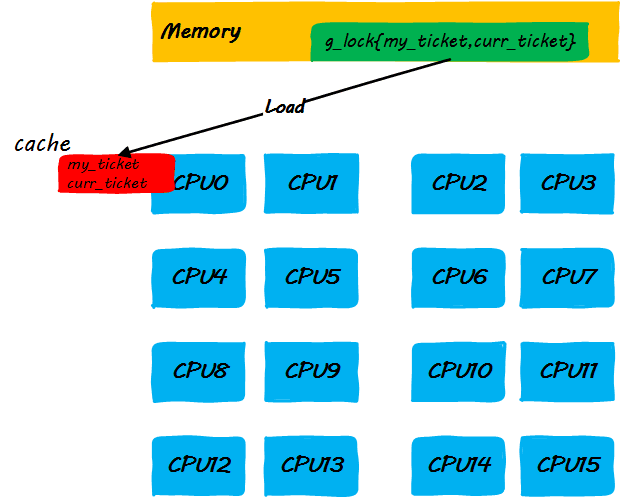• step 2：执行锁定区域指令的同时，其它CPU企图获取锁而自旋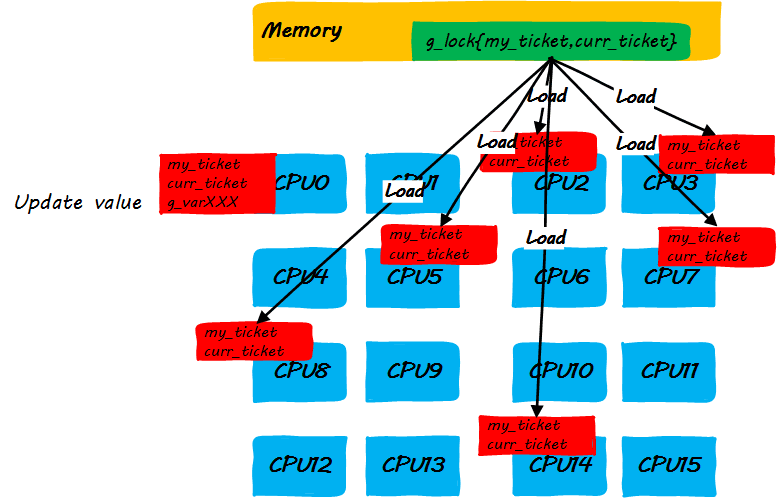• step 3：CPU0释放锁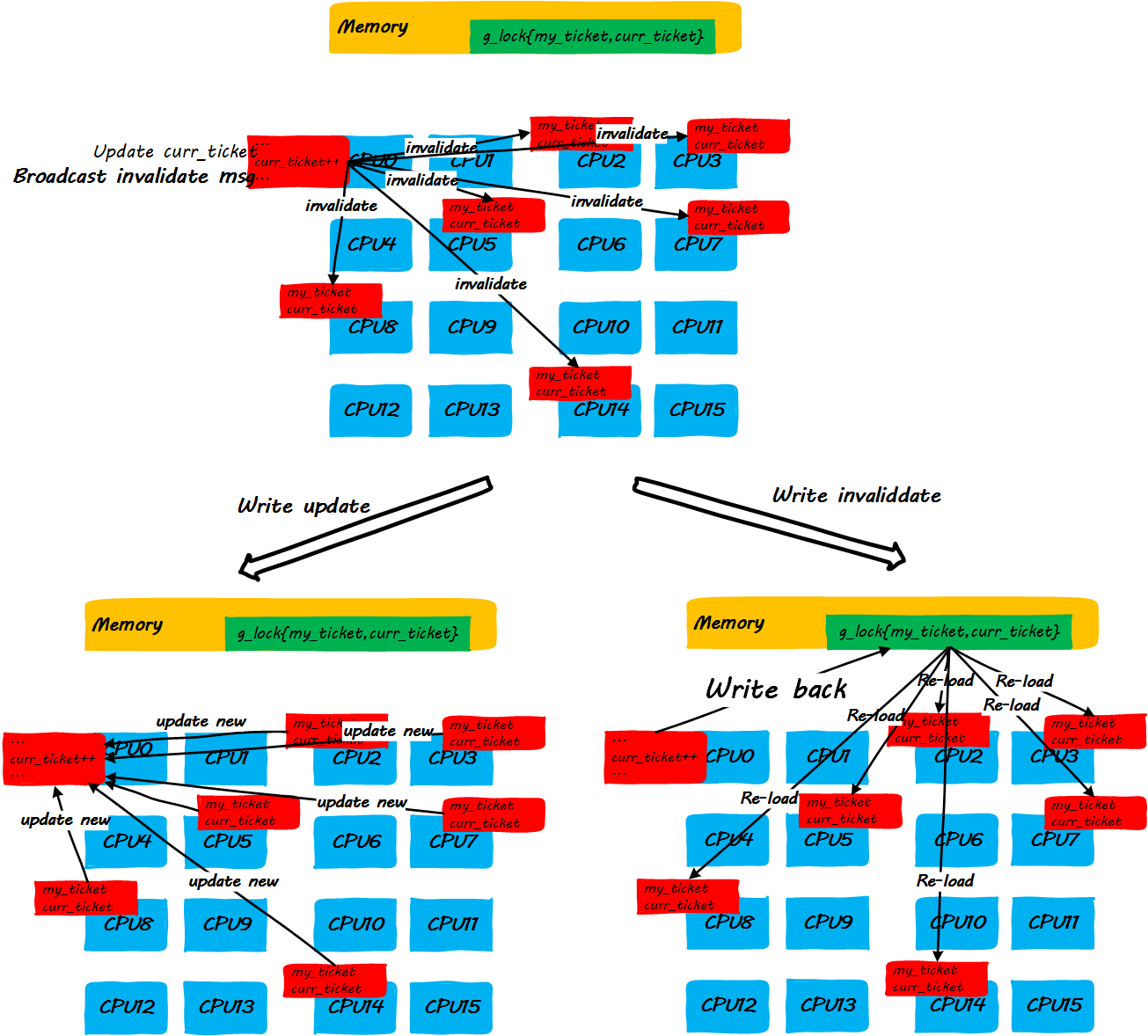## Linux spinlock的马尔科夫链模型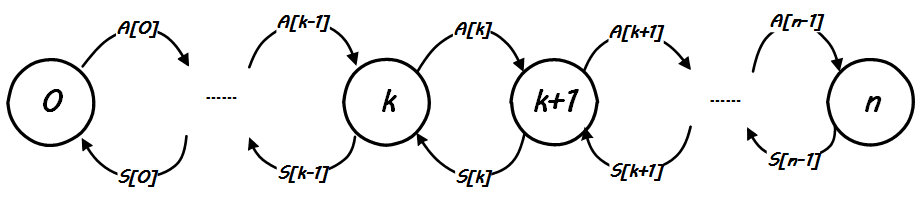$A\left[k\right]=\frac{n-k}{{T}_{arrive}}$$A[k]=\dfrac{n-k}{T_{arrive}}$

$S\left[k\right]=\frac{1}{E+\frac{k×c}{2}}$$S[k]=\dfrac{1}{E+\dfrac{k\times c}{2}}$

$设，{P}_{0},{P}_{1},...{P}_{n}是系统处在这n个状态的概率，显然\mathrm{\Sigma }{P}_{k}=1。当系统处在稳态时，下面的式子成立：$$设，P_0,P_1,...P_n是系统处在这n个状态的概率，显然\Sigma P_k=1。当系统处在稳态时，下面的式子成立：$
${P}_{k}×A\left[k\right]={P}_{k+1}×S\left[k\right]$$P_k\times A[k]=P_{k+1}\times S[k]$

${P}_{k}=\frac{\frac{1}{{T}_{arrive}^{k}\left(n-k\right)!}\prod _{i=0}^{k}\left(E+ic\right)}{\sum _{i=0}^{n}\frac{1}{{T}_{arrive}^{i}\left(n-i\right)!}\prod _{j=0}^{i}\left(E+jc\right)}$

$C=\sum _{i=0}^{n}i{P}_{i}$$C=\sum_{i=0}^{n}iP_i$

$S=x-C$$S=x-C$

#### 关于Markov chain spinlock模型的推论

$S\left[k\right]=\frac{1}{E+\frac{k×c}{2}}$$S[k]=\dfrac{1}{E+\dfrac{k\times c}{2}}$

$f\left(x\right)=\frac{1}{\alpha +x}$$f(x)=\dfrac{1}{\alpha+x}$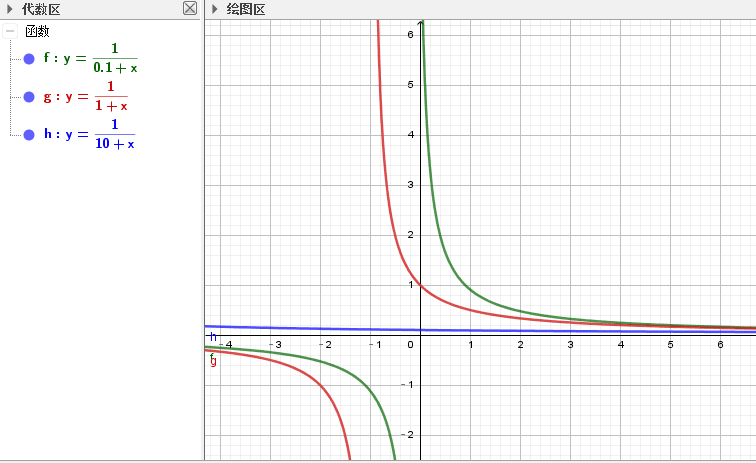## MCS spinlock如何解决问题

MCS locks and qspinlockshttps://lwn.net/Articles/590243/

OK，让我们从代码的角度来看一下为什么MCS spinlock可以解决cache一致性导致的non-scalable问题。

mcs spinlock在Linux的实现在kernel/locking/mcs_spinlock.h文件，不过直到4.14版本内核，Linux并没有哪个子系统采用这个新的spinlock，不管怎样，既然代码在手，我们便可以自己用。

• 定义MCS spinlock
struct mcs_spinlock {
struct mcs_spinlock *next; // 类似ticket的概念，所有争锁排队
int locked; /* 1 if lock acquired */ // 本地的自旋变量，不再是全局
};

• MCS spinlock上锁
// 自己的排队节点node需要自己在外部初始化，它将被排队到lock指示的等锁队列中。
void mcs_spin_lock(struct mcs_spinlock **lock, struct mcs_spinlock *node)
{
struct mcs_spinlock *prev;

/* Init node */
node->locked = 0;
node->next   = NULL;
// 原子执行*lock = node并返回原来的*lock
prev = xchg(lock, node);
if (likely(prev == NULL)) {
return;
}
// 原子执行 prev->next = node;
// 这相当于一个排入队的操作。记为(*)
WRITE_ONCE(prev->next, node);

while (0 == &node->locked)
; // 在自己的locked变量上自旋等待！
}

• MCS spinlock解锁
// 自己要传入一个排入到lock的队列中的自己的node对象node，解锁操作就是node出队并且主动将队列中下一个对象的locked帮忙设置成1.
void mcs_spin_unlock(struct mcs_spinlock **lock, struct mcs_spinlock *node)
{
// 原子获取node的下一个节点。

if (likely(!next)) {
// 二次确认真的是NULL，则返回，说明自己是最后一个，什么都不需要做。
if (likely(cmpxchg_release(lock, node, NULL) == node))
return;
// 否则说明在if判断next为MULL和cmpxchg原子操作之间有node插入，随即等待它的mcs_spin_lock调用完成，即上面mcs_spin_lock中的(*)注释那句完成以后
cpu_relax_lowlatency();
}
// 原子执行next->locked = 1;
arch_mcs_spin_unlock_contended(&next->locked);
}

void mcs_spin_lock(struct mcs_spinlock *lock);
void mcs_spin_unlock(struct mcs_spinlock *lock);

Ahha，由于同一个CPU同时只能等待一个spinlock，这件事就很简单了。来吧，让我们实现它：

struct mcs_spinlock_node {
struct mcs_spinlock_node *next;
int locked; /* 1 if lock acquired */
};

struct mcs_spinlock {
struct mcs_spinlock_node nodes[NR_CPUS];
struct mcs_spinlock_node *tail;
};

void mcs_spin_lock(struct mcs_spinlock *lock)
{
struct mcs_spinlock_node *local;
struct mcs_spinlock_node *prev;
int cpu = smp_processor_id();

local = &(lock->nodes[cpu]);
local->locked = 0;
local->next = NULL;

prev = xchg(&lock->tail, local);
if (prev == NULL) {
return;
}
WRITE_ONCE(prev->next, local);

while (!local->locked);
}

void mcs_spin_unlock(struct mcs_spinlock *lock)
{
struct mcs_spinlock_node *local;
struct mcs_spinlock_node *next;
int cpu = smp_processor_id();

cpu = smp_processor_id();
local = &(lock->nodes[cpu]);

if (likely(!next)) {
if (cmpxchg_release(lock->tail, local, NULL) == local) {
return;
}
}

local->next->locked = 1;
}

Look at this.是不是好看了很多呢？

<参考文献：MCS locks and qspinlocks>

## Aliworkqueue

aliworkqueue: Adaptive lock integration on multi-core platformhttps://patchwork.kernel.org/patch/8844841/

Wire-latency(RC delay) dominate modern computer performance,
conventional serialized works cause cache line ping-pong seriously,
the process spend lots of time and power to complete.specially on multi-core platform.
However if the serialized works are sent to one core and executed
ONLY when contention happens, that can save much time and power,
because all shared data are located in private cache of one core.
We call the mechanism as Adaptive Lock Integration.
(ali workqueue)

MaLing 离开某厂纪念文 & 介绍 aliworkqueuehttps://www.douban.com/note/596489332/

Aliworkqueue除了考虑到了spinlock本身加锁解锁对cache一致性的影响，还考虑到了spinlock保护的critical section操作的共享数据读写对cache一致性的影响，如此一来，本文上述的Markov chain模型就要进行修订了，因为critical section的操作时间不能再被当是常量$E$$E$了，而它也要作为一个和CPU核数相关的量参与到模型的构建中了。

## 后记

©️2019 CSDN 皮肤主题: 编程工作室 设计师: CSDN官方博客